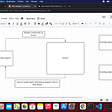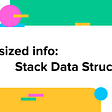# What Is Big-O programming?

Big-O programing wow, sounds scary right. Well, I’ve got good news for you Big-O is actually very simple to understand. To put it simply Big-O is just a way of talking about the efficiency of Algorithms. Lemme give you an example of Big-O Notation.

`let number = 10`

The Big-O of this would be just be `O(1)`. Why `O(1)`? let me explain in further detail. `O = the number of operations in a given function.` Since our code above will only run once it is equal to 1 operation. Pretty simple right. Okay well, let's take it one step further.

`const myArray = ["a", "b", "c", "d"]const findCharacter = (char, array) => {    for(let i = 0; i < array.length; i++){        if(array[i] === char){            return true        }    }    return false}`

What do you think the Big-O of `findCharacter` will be? What does the function do?

`const myArray = ["a", "b", "c", "d"]//we already know that this will be O(1) because it will only run once.//here we say. for each elemant in the array check to see if char exsits, if it does stop running and return true.//if none of the characters return true then we'll finish the loop and return false.const findCharacter = (char, array) => {    for(let i = 0; i < array.length; i++){ // the number of operations is equal to the size of the array.        if(array[i] === char){            return true        }    }    return false}myArray.length//=> 4//if the size of the array is 4 and that means the total number of operations would depend on the size of our array.`

So since the `n` the number of operations depends on the size of the array or data we would of `O(n)`. where `n` represents our data. you could say that it is also `O(4)`. Since the array has 4 elements the number of operations will also be four. let's look at another example.

`//compare to arraysconst array1 = ["a", "b", "c", "d",]const array2 = ["x", "y", "z", "c"]//we want to check if any of that character in array1 are also in array two.//I'm justing going to code out the brute force solution first.const compareArrays = (arr1, arr2) => {    for(let i = 0; i < arr1.length; i++){        for(let j = 0; j < arr2.length; j++){            if(arr1[i] === arr2[j]){                return true            }        }    }    return false}`

This is a bit of a tricky one. If we say that bot `array1` and `array2` will always have the same size then we’d get `O(n^2)`. The reason for this is because our first array `n` will be the same length as our second array. Lemme walk you through it a little more.

`const compareArrays = (arr1, arr2) => { for(let i = 0; i < arr1.length; i++){// the array is length is 4    for(let j = 0; j < arr2.length; j++){ //and for every item in array2 which is 4 run the code 4 times.     if(arr1[i] === arr2[j]){ //4*4 16 and 4^2 is also 16 therfore we get O(n^2)       return true      }    }  } return false}`

Pretty simple right. but if the arrays had different lengths then the bigO would be `O(n*b)` let's say array1 has a length of two and array1 has a length of 10. Then if we plugin those values, the number of operations is `2*10` we loop through array1 twice and for every element in array1 we loop through array2 ten times. The total number of operations would now be twenty and that is why we note it as `O(n*b)` where `n` is the length of the data for array1 and `b` is the length of data for array2.

Big-O may seem scary but it is really simple once you understand what it is and why we use it.

--

--

--

## More from Nerd For Tech

NFT is an Educational Media House. Our mission is to bring the invaluable knowledge and experiences of experts from all over the world to the novice. To know more about us, visit https://www.nerdfortech.org/.

## Let’s talk about React and MVP## News app using Account kit, Ads kit, and Push kit with HMS Core## How To Add Swagger To NodeJS REST API## Headless CMS: Enable In-Context Preview and Editing in an External Application## Implementing Javascript Array class methods.## How To Build CI/CD For Static Next.js App Using Azure DevOps## Multi-player Online feature (part 4— creating newly connected player’s sprite and deleting…## Rendering JSX From Strings## Cameron J. Leverett

I love tackling new and exciting challenges and working with software because it’s consistently changing, which is exciting.

## Byte size info: Stack data Structures## My experience of being a self-taught software developer## 100 JavaScript Interview Questions & Answers in 2022